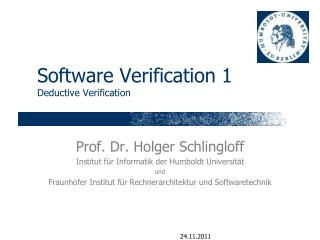Download PresentationSoftware Verification 1 Deductive Verification# Software Verification 1 Deductive Verification - PowerPoint PPT Presentation

Download Presentation##### Software Verification 1 Deductive Verification

Download Policy: Content on the Website is provided to you AS IS for your information and personal use and may not be sold / licensed / shared on other websites without getting consent from its author. While downloading, if for some reason you are not able to download a presentation, the publisher may have deleted the file from their server.

- - - - - - - - - - - - - - - - - - - - - - - - - - - E N D - - - - - - - - - - - - - - - - - - - - - - - - - - -
##### Presentation Transcript

1. Software Verification 1Deductive Verification Prof. Dr. Holger Schlingloff Institut für Informatik der Humboldt Universität und Fraunhofer Institut für Rechnerarchitektur und Softwaretechnik

2. Structured Operational Semantics • Denotational semantics can be made mathematically sound, but is not “intuitive” • Operations of a “real” machine? • transitions from valuation to valuation • program counter is increased with the program • Abstract representation: • state=(program, valuation) • program means the part which is still to be executed • transition=(state1, state2) • “Meaning” of a program is a (possibly infinite) set of such transitions

3. SOS-Rules • (v=t, V)(skip, V[v:=t]); • ({skip; },V) (,V) • if (1, V1) (2,V2), then ({1; }, V1) ({2; },V2) • if (U,I,V) ⊨ b, then (if (b) 1 else 2, V) (1,V) • if (U,I,V) ⊭ b, then (if (b) 1 else 2, V) (2,V) • (while (b) , V) (if (b) {; while (b) }}, V) • these are so-called “small-step rules”; “big-step rule”: if (1, V1) (2,V2), and (2, V2) (3,V3), then ({1; 2}, V1) (3, V3) • derivable?

4. SOS-Example • (while (a!=0) {c = a; a = b%a; b = c},(a=20, b=12, c=0)) ...

5. About operational semantics • For every (1, V1), there is exactly one sequence(1, V1)(2, V2)(3, V3) ... • allows to “symbolically execute” a program • does not allow to show properties • e.g. “program calculates gcd” • e.g. “program terminates” • Hoare-Tripel: {}  {}meaning: if  holds before the execution of , then  holds afterwards •  and  are first-order formulas (possibly with quantification; logical variables vs. program variables)

6. Hoare calculus • ⊢ {[v:=t]} v=t {} (ass) • ⊢ {} skip {} (usually omitted) • if ⊢ {}1{}and ⊢ {} 2{}, then {} {1; 2}{} (seq) • if ⊢ {  b} 1{} and ⊢ {  ¬b} 2{}, then ⊢ {}if (b) 1 else 2 {} (ite) • if ⊢ {  b} {}, then ⊢ {}while (b) {  ¬b} (whi) • If ⊢ (’  ) and ⊢ {} {}, then ⊢ {’}  {} (imp1) • If ⊢ {}  {} and ⊢ (  ’), then ⊢ {}  {’} (imp2) • the semantics (meaning) of a program  is the set of all derivable Hoare-tripels {} {}

7. Examples • {x==17} x++ {x==18} • {x==17} y=x+1 {y==18} • {x==17} {x++; y=x+1} {y==19} • {a==m  b==n}if (a<=b) c = a else c = b{c==min(m,n)} • {a==m>0  b==n>0} while (a!=0) {c = a; a = b%a; b = c} {b==gcd(m,n)}